Confidence Intervals

# Introduction

Have you ever wondered what the average number of M&Ms in a bag at the grocery store is? You can use confidence intervals to answer this question. (credit: comedy_nose/flickr)Chapter Objectives

By the end of this chapter, the student should be able to:

• Calculate and interpret confidence intervals for estimating a population mean and a population proportion.
• Interpret the Student’s t probability distribution as the sample size changes.
• Discriminate between problems applying the normal and the Student’s t distributions.
• Calculate the sample size required to estimate a population mean and a population proportion given a desired confidence level and margin of error.

Suppose you were trying to determine the mean rent of a two-bedroom apartment in your town. You might look in the classified section of the newspaper, write down several rents listed, and average them together. You would have obtained a point estimate of the true mean. If you are trying to determine the percentage of times you make a basket when shooting a basketball, you might count the number of shots you make and divide that by the number of shots you attempted. In this case, you would have obtained a point estimate for the true proportion.

We use sample data to make generalizations about an unknown population. This part of statistics is called inferential statistics. The sample data help us to make an estimate of a population parameter. We realize that the point estimate is most likely not the exact value of the population parameter, but close to it. After calculating point estimates, we construct interval estimates, called confidence intervals.

In this chapter, you will learn to construct and interpret confidence intervals. You will also learn a new distribution, the Student’s-t, and how it is used with these intervals. Throughout the chapter, it is important to keep in mind that the confidence interval is a random variable. It is the population parameter that is fixed.

If you worked in the marketing department of an entertainment company, you might be interested in the mean number of songs a consumer downloads a month from iTunes. If so, you could conduct a survey and calculate the sample mean,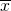, and the sample standard deviation, s. You would useto estimate the population mean and s to estimate the population standard deviation. The sample mean,, is the point estimate for the population mean, μ. The sample standard deviation, s, is the point estimate for the population standard deviation, σ.

Each ofand s is called a statistic.

A confidence interval is another type of estimate but, instead of being just one number, it is an interval of numbers. It provides a range of reasonable values in which we expect the population parameter to fall. There is no guarantee that a given confidence interval does capture the parameter, but there is a predictable probability of success.

Suppose, for the iTunes example, we do not know the population mean μ, but we do know that the population standard deviation is σ = 1 and our sample size is 100. Then, by the central limit theorem, the standard deviation for the sample mean is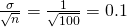.

The empirical rule, which applies to bell-shaped distributions, says that in approximately 95% of the samples, the sample mean,, will be within two standard deviations of the population mean μ. For our iTunes example, two standard deviations is (2)(0.1) = 0.2. The sample meanis likely to be within 0.2 units of μ.

Becauseis within 0.2 units of μ, which is unknown, then μ is likely to be within 0.2 units ofin 95% of the samples. The population mean μ is contained in an interval whose lower number is calculated by taking the sample mean and subtracting two standard deviations (2)(0.1) and whose upper number is calculated by taking the sample mean and adding two standard deviations. In other words, μ is between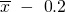and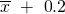in 95% of all the samples.

For the iTunes example, suppose that a sample produced a sample mean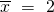. Then the unknown population mean μ is between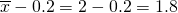and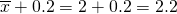We say that we are 95% confident that the unknown population mean number of songs downloaded from iTunes per month is between 1.8 and 2.2. The 95% confidence interval is (1.8, 2.2).

The 95% confidence interval implies two possibilities. Either the interval (1.8, 2.2) contains the true mean μ or our sample produced anthat is not within 0.2 units of the true mean μ. The second possibility happens for only 5% of all the samples (95–100%).

Remember that a confidence interval is created for an unknown population parameter like the population mean, μ. Confidence intervals for some parameters have the form:

(point estimate – margin of error, point estimate + margin of error)

The margin of error depends on the confidence level or percentage of confidence and the standard error of the mean.

When you read newspapers and journals, some reports will use the phrase “margin of error.” Other reports will not use that phrase, but include a confidence interval as the point estimate plus or minus the margin of error. These are two ways of expressing the same concept.

Note

Although the text only covers symmetrical confidence intervals, there are non-symmetrical confidence intervals (for example, a confidence interval for the standard deviation).

Collaborative Exercise

Have your instructor record the number of meals each student in your class eats out in a week. Assume that the standard deviation is known to be three meals. Construct an approximate 95% confidence interval for the true mean number of meals students eat out each week.

1. Calculate the sample mean.
2. Let σ = 3 and n = the number of students surveyed.
3. Construct the interval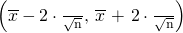.

We say we are approximately 95% confident that the true mean number of meals that students eat out in a week is between __________ and ___________.

### Glossary

Confidence Interval (CI)
an interval estimate for an unknown population parameter. This depends on:

• the desired confidence level,
• information that is known about the distribution (for example, known standard deviation),
• the sample and its size.
Inferential Statistics
also called statistical inference or inductive statistics; this facet of statistics deals with estimating a population parameter based on a sample statistic. For example, if four out of the 100 calculators sampled are defective we might infer that four percent of the production is defective.
Parameter
a numerical characteristic of a population
Point Estimate
a single number computed from a sample and used to estimate a population parameter